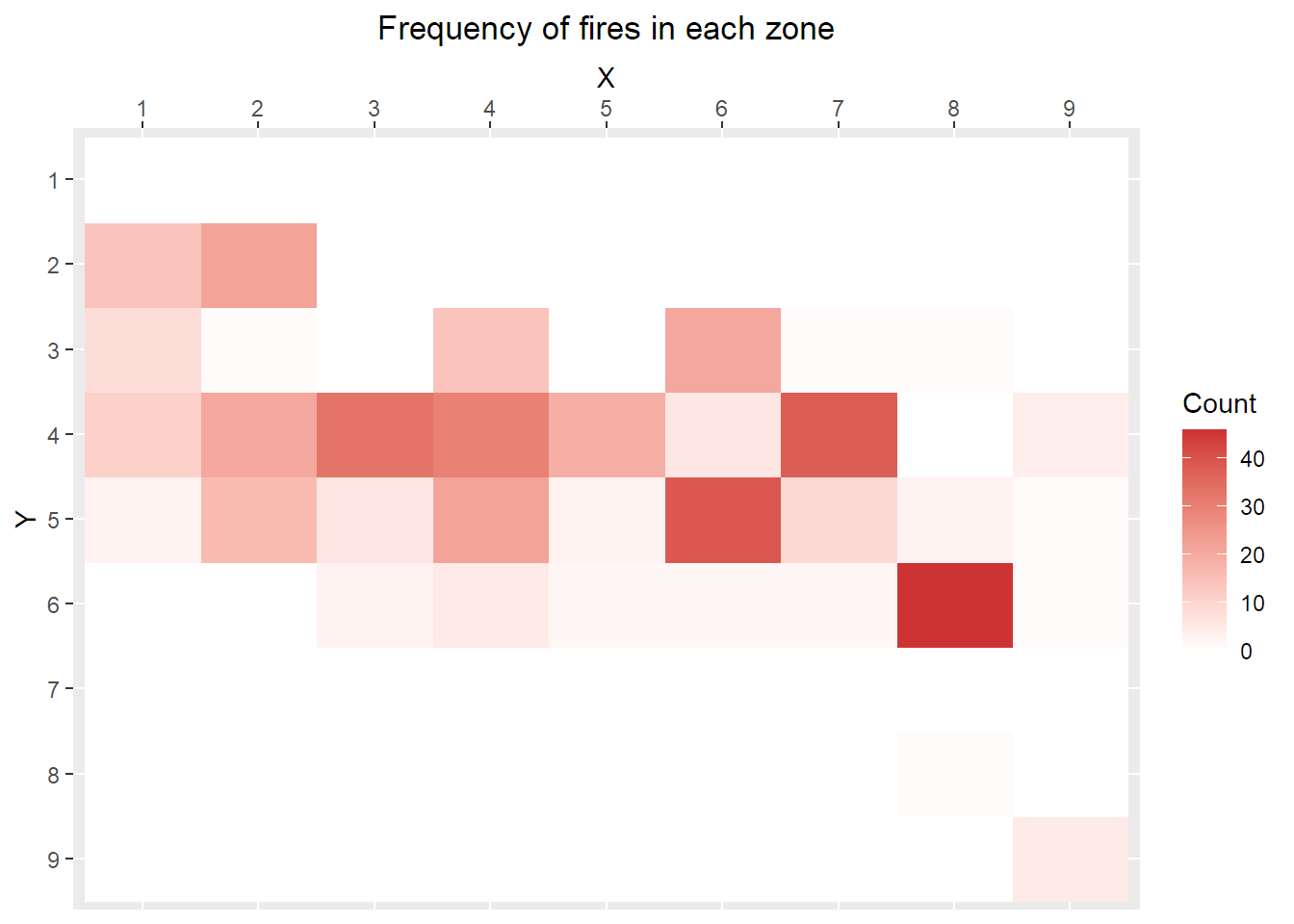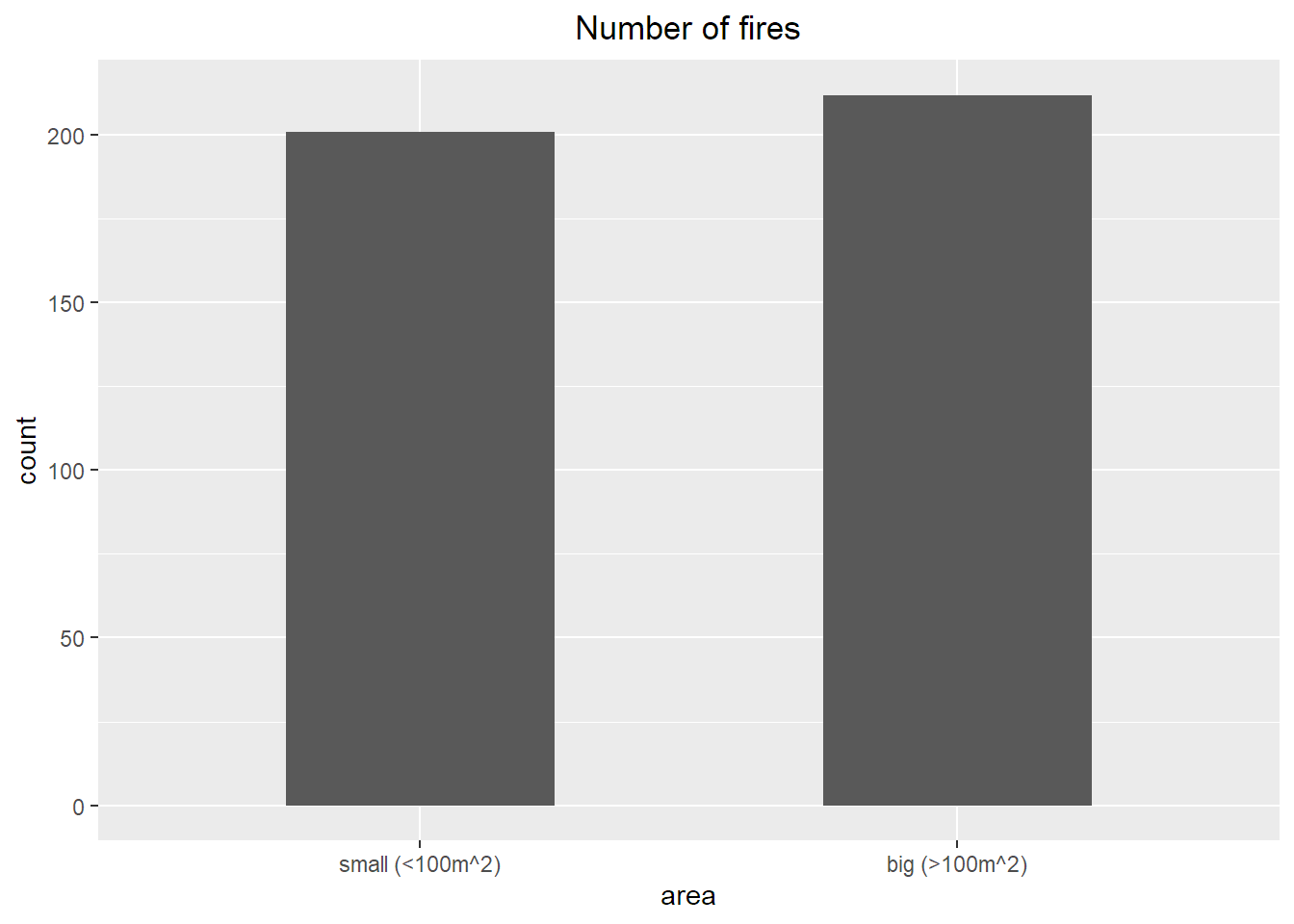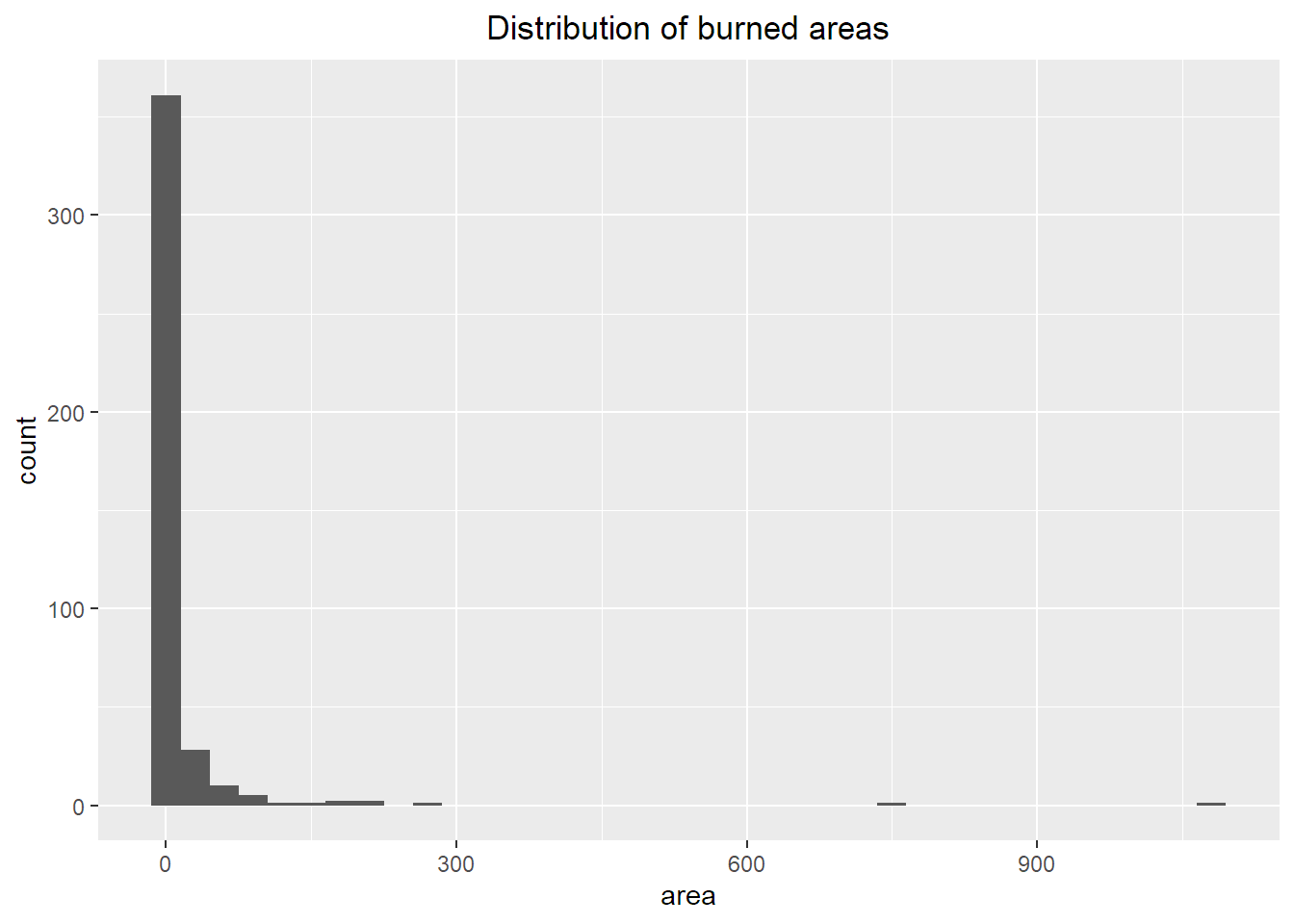# 1 Introduction

Forest fires are a major environmental concern. Wildfires cause environmental and economical damage, destroy large areas of land and displace entire communities. Forest ecosystems could once easily bounce back from natural occurring fires. However, due to climate change, this is no longer the case, as longer periods of drought and rising temperatures do not allow forests to recover. Therefore, it is an increasingly important concern to prevent wildfires or, at least, limit the damage they cause by detecting their occurrence as soon as possible.

Our goal is to predict the burned area of forest fires, measured in hectares (ha). There are various techniques to detect forest fires, such as satellite systems and infrared scanners. However, the deployment of these systems is costly and their response may be delayed by various factors. Another possibility is to analyze weather conditions such as humidity and temperature, which are known to affect fire occurrences. Unlike other systems, weather data may be easily collected in real time employing cheap sensors. We will use this kind of readily accessible data for our prediction task.

library(tidyverse)
library(ggplot2)
library(ggcorrplot)
library(cowplot)
library(tree)
library(ranger)
library(caret)

# Set categorical variables as factors
fires.data$X <- factor(fires.data$X)
fires.data$Y <- factor(fires.data$Y)
fires.data$month <- factor(fires.data$month, levels=c("jan", "feb", "mar", "apr", "may", "jun", "jul", "aug", "sep", "oct", "nov", "dec"))
fires.data$day <- factor(fires.data$day, levels=c("mon", "tue", "wed", "thu", "fri", "sat", "sun"))

# Settings for plots
point_alpha <- 0.4
line_color <- "brown3"

# 2 Dataset

The data consists of a collection of 517 records, each corresponding to a fire occurred in the Montesinho natural park, in the northeast region of Portugal, from January 2000 to December 2003. Each record presents 12 attributes, including spatial data, temporal data, information regarding weather conditions and weather indices. Additionally, each record contains the value of the area burned by the forest fire, which is our target for the regression task. The dataset does not contain any missing value.

fires.data

## 2.1 Source

The dataset was created by by manually merging two datasets. The first one was provided by an inspector that was responsible for the Montesinho fire occurrences, who collected temporal data and spatial data for each fire, along with the components of the FWI system and the total burned area. The second one was collected by the Polytechnic Institute of Bragança, and contains several weather observations that were recorded within a 30 minute period by a meteorological station located at the center of the park.

## 2.2 Attributes

The following table describes the dataset attributes.

Attribute Description Type
X X-axis spatial coordinate within the Montesinho park map Qualitative (factor) with 9 levels
Y Y-axis spatial coordinate within the Montesinho park map Qualitative (factor) with 7 levels
month Month of the year Qualitative (factor) with 12 levels
day Day of the week Qualitative (factor) with 7 levels
FFMC FFMC index from the FWI system Quantitative
DMC DMC index from the FWI system Quantitative
DC DC index from the FWI system Quantitative
ISI ISI index from the FWI system Quantitative
temp Temperature in Celsius degrees Quantitative
RH Relative humidity in % Quantitative
wind Wind speed in km/h Quantitative
rain Outside rain in mm/m² Quantitative
area Burned area of forest in ha Quantitative

## 2.3 Training and testing

Our goal is to build a model that is capable of predicting the burned area of forest fires, given a set of predictors. To test the generalization ability of our models, we hold out part of the available data as a test set. This set is useful to evaluate the prediction performance of a model on unseen data.

To generate a test set, we randomly sample 20% of our dataset. The remaining 80% is regarded as the training set, i.e., the data we use to build and validate our models.

train_nrow <- floor(0.8 * nrow(fires.data))

set.seed(42)  # For reproducibility
train_idx <- sample(seq_len(nrow(fires.data)), size=train_nrow)

fires <- fires.data[train_idx, ]
cat("Training set size:", nrow(fires))
## Training set size: 413
fires.test <- fires.data[-train_idx, ]
cat("Test set size:", nrow(fires.test))
## Test set size: 104

Note that exploratory data analysis is performed exclusively on the training set, to avoid any information leakage during data preprocessing steps.

## 2.4 Exploratory data analysis

### 2.4.1 Spatial data

The position of the fires is encoded into a 9 by 9 grid, superimposed over the Montesinho park map. The following heatmap shows how many fires occurred at each (X, Y) coordinate pair. It is evident, according to this data, that the position influences the probability of fire occurrence.

coord_counts <- merge(as.data.frame(table(fires[, 1:2])), expand.grid(X=as.factor(c(1:9)), Y=as.factor(c(1:9))), by=c("X", "Y"), all=TRUE)

ggplot() +
geom_raster(data=coord_counts, aes(x=X, y=Y, fill=Freq)) +
scale_fill_gradient(low="white", high="brown3", na.value = "white", name="Count") +
scale_x_discrete(position = "top") +
scale_y_discrete(limits=factor(9:1)) +
ggtitle("Frequency of fires in each zone") +
theme(plot.title = element_text(hjust = 0.5))### 2.4.2 Burned area

Our prediction target is the area burned by the forest fire. One important peculiarity of this dataset is that burned areas smaller than $$1/100\; \text{ha} = 100\; \text{m}^2$$ are marked as $$0$$. The following plot shows the number of small fires against bigger fires.

small_big_count <- data.frame(
factor(c("small (<100m^2)", "big (>100m^2)"), levels=c("small (<100m^2)", "big (>100m^2)")),
c(sum(fires$area == 0), sum(fires$area > 0))
)

colnames(small_big_count) <- c("area", "count")

ggplot(data=small_big_count, aes(x=area, y=count)) +
geom_bar(stat="identity", width=0.5) +
ggtitle("Number of fires") +
theme(plot.title = element_text(hjust = 0.5))The dataset contains a similar amount of big and small fires. It is necessary to keep this distinction in mind going forward.

The following histogram shows the burned area distribution of the training set. As we can see, even if we only consider fires larger than the threshold, the distribution is still heavily right-skewed. Moreover, the data presents a few outliers much larger than the others.

ggplot() +
geom_histogram(data=fires, mapping=aes(x=area), binwidth=30) +
ggtitle("Distribution of burned areas") +
theme(plot.title = element_text(hjust = 0.5))The authors of the dataset suggest to apply a transformation to the area attribute, namely $$\ln{(x+1)}$$, to reduce skewness and improve symmetry of the distribution of the target variable. The following plot shows the distribution of the burned area after this transformation. Note that our models will employ the transformed target rather than the original one.

ggplot() +
geom_histogram(data=fires, mapping=aes(x=log(area+1)), binwidth=0.2) +
ggtitle("Distribution of burned areas (log)") +
theme(plot.title = element_text(hjust = 0.5))